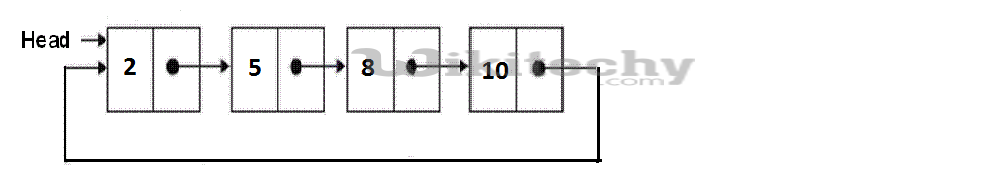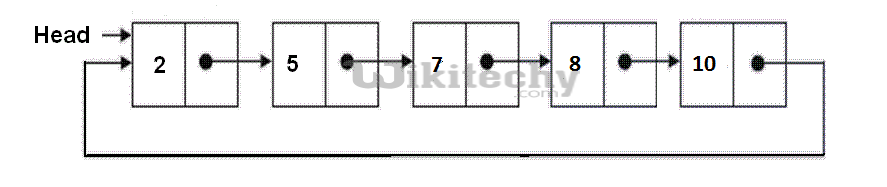# python Algorithm-Sorted insert for circular linked list

Sorted insert for circular linked list - Circular linked list - Write a C function to insert a new value in a sorted Circular Linked List.

Write a C function to insert a new value in a sorted Circular Linked List (CLL). For example, if the input CLL is following:After insertion of 7, the above CLL should be changed to followingAlgorithm:
Allocate memory for the newly inserted node and put data in the newly allocated node. Let the pointer to the new node be new_node. After memory allocation, following are the three cases that need to be handled.

```1) Linked List is empty:
a)  since new_node is the only node in CLL, make a self loop.
new_node->next = new_node;
b) change the head pointer to point to new node.
2) New node is to be inserted just before the head node:
(a) Find out the last node using a loop.
current = current->next;
(b) Change the next of last node.
current->next = new_node;
(c) Change next of new node to point to head.
(d) change the head pointer to point to new node.
3) New node is to be  inserted somewhere after the head:
(a) Locate the node after which new node is to be inserted.
current->next->data < new_node->data)
{   current = current->next;   }
(b) Make next of new_node as next of the located pointer
new_node->next = current->next;
(c) Change the next of the located pointer
current->next = new_node;```

Python Programming:

``````# Node class
class Node:

# Constructor to initialize the node object
def __init__(self, data):
self.data = data
self.next = None

def __init__(self):

# Function to insert a new node at the beginning
def push(self, new_data):
new_node = Node(new_data)

def printList(self):
print temp.data,
temp = temp.next
print temp.data,
temp = temp.next

""" function to insert a new_node in a list in sorted way.
Note that this function expects a pointer to head node
as this can modify the head of the input linked list """
def sortedInsert(self, new_node):

# Case 1 of the above algo
if current is None:
new_node.next = new_node

# Case 2 of the above algo
elif (current.data >= new_node.data):

# If value is smaller than head's value then we
# need to change next of last node
current = current.next
current.next = new_node

# Case 3 of the above algo
else:

# Locate the node before the point of insertion
current.next.data < new_node.data):
current = current.next

new_node.next = current.next
current.next = new_node

# Driver program to test the above function
arr = [12, 56, 2, 11, 1, 90]

list_size = len(arr)

# Create linked list from the array arr[]
# Created linked list will be 1->2->11->12->56->90
for i in range(list_size):
temp = Node(arr[i])
start.sortedInsert(temp)

start.printList()``````

READ  Java Algorithm - Find the middle of a given linked list

Output:

`1 2 11 12 56 90`

Time Complexity: O(n) where n is the number of nodes in the given linked list.

Case 2 of the above algorithm/code can be optimized. Please see this comment from Pavan. To implement the suggested change we need to modify the case 2 to following.

``````// Case 2 of the above algo
else if (current->data >= new_node->data)
{
// swap the data part of head node and new node
// assuming that we have a function swap(int *, int *)
swap(&(current->data), &(new_node->data));

}``````#### Venkatesan Prabu

Wikitechy Founder, Author, International Speaker, and Job Consultant. My role as the CEO of Wikitechy, I help businesses build their next generation digital platforms and help with their product innovation and growth strategy. I'm a frequent speaker at tech conferences and events.

X